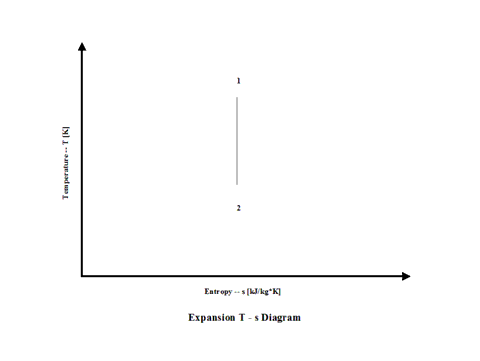top of page

### Expansion Analysis

Expansion

Introduction

This section provides an isentropic expansion analysis when the working fluid is air.

Analysis

In the presented expansion analysis, only air is considered as the working fluid behaving as a perfect gas -- specific heat has a constant value.  Ideal gas state equation is valid -- pv = RT.

Air enters a turbine at point 1 and it exits the turbine at point 2.  Isentropic expansion is considered with no entropy change.

Figure 1 presents an expansion schematic layout.Figure 1 - Expansion Schematic Layout

Figure 2 presents an expansion temperature vs entropy diagram.Figure 2 - Expansion Temperature vs Entropy Diagram

Figure 3 presents expansion specific power output values for a few typical expansion ratio values.  It should be noted that the air enters the turbine at the temperature of 1,500 [K] and the turbine exhaust pressure is always equal to the standard ambient pressure -- 1 [atm] of absolute pressure.Figure 3 - Expansion Specific Power Output

Figure 4 presents expansion power output values for two typical expansion ratio values and a few different working fluid mass flow rate values for a fixed expansion inlet temperature.Figure 4 - Expansion Power Output

One can notice that both expansion specific power output and power output increase with an increase in the expansion ratio values.  As the working fluid mass flow rate increases for a fixed expansion inlet temperature, the expansion power output values increase too.

Assumptions

Working fluid is air.  There is no friction and heat transfer. Expansion is isentropic -- there is no entropy change.  Ideal gas state equation is valid -- pv = RT. Air behaves as a perfect gas -- specific heat has a constant value.

Governing Equations

T1/T2 = (p1/p2)^(ϰ-1)/ϰ
ϰ = cp/cv
cp - cv = R
pv = RT
w = c
p(T1 - T2)
W = c
p(T1 - T2)m

Input Data

T1 = 1,500 [K]
p
1 = 5, 10 and 15 [atm]
p
2 = 1 [atm]
R = 0.2867 [kJ/kg*K]
c
p = 1.004 [kJ/kg*K]

ϰ = 1.4 [/]
m = 50, 100 and 150 [kg/s]

Results

Specific Power Output vs Expansion Ratio
Expansion Inlet Temperature = 1,500 [K]

Compression
Ratio
[/]

5

10

15

Specific Power
Input
[kW/kg/s]

174

278

349

Power Output vs Expansion Ratio for a few Mass Flow Rates
Expansion Inlet Temperature = 1,500 [K]

Power Output
[MW]

Compression Ratio
[/]

5

15

Mass Flow Rate
[kg/s]

50

27.8

40.6

100

55.5

81.1

150

83.3

121.7

Conclusions

Both expansion specific power output and power output increase with an increase in the expansion ratio values.  As the working fluid mass flow rate increases for a fixed expansion inlet temperature, the expansion power output values increase too.

References

JANAF Thermochemical Data - Tables, 1970

bottom of page